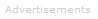Standard addition is used in quantitative analysis of chemicals. It is a calibration method that involves adding an additional amount of the substance of interest to a sample when a one-off or infrequently performed analysis is required.An analyst usually divides the unknown sample into two portions, so that a known amount of the analyte (a spike) can be added to one portion. These two samples, the original and the original plus spike, are then analyzed. The sample with the spike will show a larger analytical response than the original sample due to the additional amount of analyte added to it.

The difference in analytical response between the spiked and unspiked samples is due to the amount of analyte in the spike. This provides a calibration point to determine the analyte concentration in the original sample.

The procedure is suitable when, for example, due to matrix effects the analytical response for an analyte in a complex sample may not be the same as for the analyte in a simple standard. In this case, calibration with a working curve would require standards that closely match the composition of the sample.

For routine analyses it is feasible to prepare or purchase realistic standards, e.g. NIST standard reference materials. For diverse and one-of-a-kind samples, however, this procedure is time consuming and often impossible.

An alternative calibration procedure is the standard addition method as outlined at the beginning of the page.

Example Calculation: The Fluoride Ion-Selective Electrode

Two 10.0 ml aliquots are taken from a sample of wastewater effluent and one is spiked with 1.00 ml of 0.0207 M NaF standard solution.

The response from a F--selective electrode is measured to be 66.2 mV for the unspiked sample and 28.6 mV for the spiked sample.

Note: we work with the fluoride concentration [F- ] for clarity and simplicity, but the electrode actually measures activity.

The F- concentration in the original sample is:

E = constant - 2.303 * (RT/nF) * log[F-]

Measurement of the unspiked sample:

0.0662 V = constant - (0.0591 V) * log[F-]

Measurement of the spiked sample:

0.0286 V = constant - (0.0591 V) * log{([F-]0.0100 L + 0.0207 M * 0.00100 L)/0.0110 L}

0.0286 V = constant - (0.0591 V) * log{0.909[F-] + 0.00188}

Combining equations:

0.0662 V - 0.0286 V = -(0.0591 V) * log{[F-]/(0.909[F-] + 0.00188)}

-0.636 = log{[F-]/(0.909[F-] + 0.00188)}

0.231 = [F-]/(0.909[F-] + 0.00188)

[F-] = 5.50 x 10-4 MSearch the Dictionary for More Terms